﻿ Top 5 Machine Learning Algorithms You Need to Know

# Top 5 Machine Learning Algorithms You Need to Know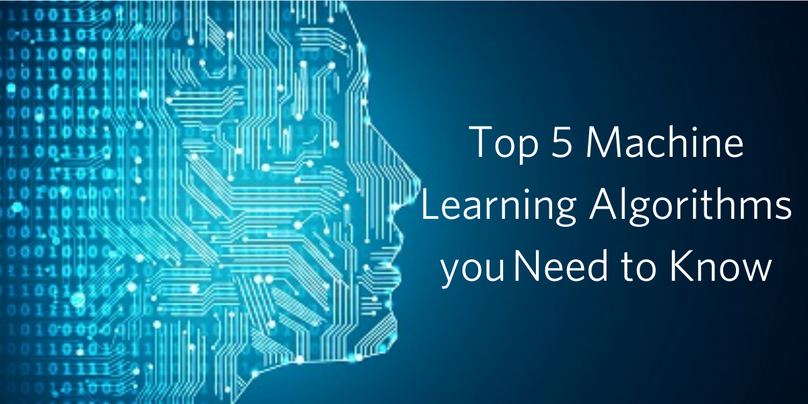Understanding algorithms can make our lives as developers easier, so it's been frustrating to personally find most machine learning tutorials to either be too high-level or too low-level to be beginner-friendly. This blog post is meant to make machine learning algorithms accessible to all, including non-ML engineers.

• Supervised learning: model learns by looking at correctly-classified, already-labeled data
• Unsupervised learning: model learns by looking at and trying to group unlabeled data according to similarities, patterns and differences on its own

### Supervised learning can solve these problems

• Classification: predicts a discrete label or category, like "red", "yes", "pie", "fruit" (ie. will this house sell, what color will the sunset be).
• Regression: predicts a continuous value or quantity, like currency, miles, people . (ie. how much will this house sell for, what time will the sun set).

Here are some supervised learning algorithms which you will come across in ML.

### 5 Algorithms to Know

1. Linear Regression is one of the most commonly-used ML algorithms and statistics techniques. It explains the relationship between

1. one or more independent predictor variables (the x-values)
2. one explanatory dependent numerical outcome variable (the y-values)

by fitting a linear equation to observed data which can then be used to predict future values.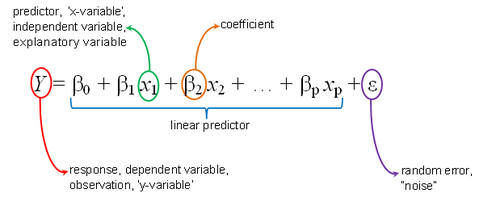(source)

The most popular technique for linear regression, least squares, calculates the best-fitting line (also known as regression line) for when there is only one independent variable. Its formula is y = mx + b, such that the line is the closest it can be to each data point.

You may use a linear regression model if you wanted to examine the relationship between someone's weight and height or their experience and salary or, given known data, predict how many people will get COVID-19 next, etc.

2. Logistic Regression is similar to linear regression, but is a binary classifier algorithm (it assigns a class to a given input, like saying an image of a pie is a "pie" or a "cake" or someone will come in 1st, 2nd, 3rd, 4th place) used to predict the probability of an event occurring given data. It works with binary data and is meant to predict a categorical "fit" (one being success and zero being failure, with probabilities in between), whereas Linear Regression's result could have infinite values and predict a value with a straight line. Logistic regression instead produces a logistic curve constrained to values between zero and one to examine the relationship between the variables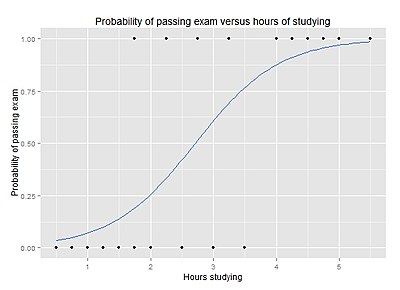(source)

You may use a logistic regression model to determine if someone will win an election or game, whether or not you have COVID-19, if someone will pass an interview or fail it, if a customer will come back, if a phone call is spam, etc.

 Linear Regression Logistic Regression Regression algorithm Regression algorithm forced to output classification given binary data Result (dependent variable) has continuous, infinite number of possible values: weight, height, number of hours, etc. Result (dependent variable is binary) is a categorical probability of success and has a limited number of possible values Algorithm results in a line (Y = mX + b). Algorithm results in a logistic curve whose y-values must be between 0 and 1 and whose sum adds to 1

3. Naive Bayes is a family of supervised classification algorithms that calculate conditional probabilities. They're based on Bayes’ Theorem which, assuming the presence of a particular feature in a class is independent of the presence of other features, finds a probability when other probabilities are known.

For example, you could say a sphere is a tennis ball if it is yellow, small, and fuzzy. Even if these features depend on one another or upon the existence of another, each of these features independently contribute to the probability that this sphere is a tennis ball. This is why the algorithm is called "naive"-- it assumes each feature is independent.

Once the algorithm has gone through each feature and calculated conditional probability, it makes a decision about the predicted class, providing a binary outcome in classification problems.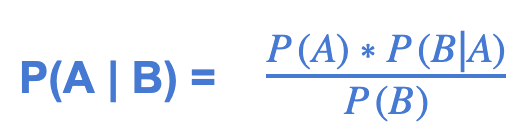Say A is an animal, and B are observations you make about the animal.

A = dog and B = it is furry, has a tail, and has 4 legs:

•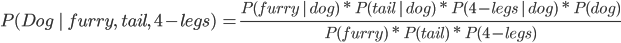•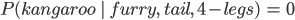* P(kangaroo | furry, tail, and 4 legs) = 0 because P(4 legs | kangaroo) = 0

With this equation you could find the probability A happens given the occurrence of B where B is the evidence and A is the hypothesis.

You may use Naive Bayes for real-time prediction (it’s fast), multi-class prediction, robotics, computer vision, and natural language processing tasks like text classification, spam filtering, sentiment analysis, and recommendations.

So what's the difference between logistic regression and Naive Bayes? Because Naive Bayes is naive, the algorithm expects the features to be independent which is not always true, so its prediction would be off then. Logistic regression works better if the features are not all independent of each other.

4. KNN (K-nearest neighbors) is a supervised algorithm to solve both classification and regression predictive problems. It assumes similar values are close to each other, like data points on a graph. Given a new unclassified data point, the algorithm finds k neighbors of this new value from an existing dataset with some distance function (like Euclidean, Manhattan, or Minkowski) and returns a prediction of the most common outcome by calculating the average of k number of neighbors.

Say the new blue point below is added to the dataset and you want to classify it. You would calculate the distance between the blue point and each other point, searching for the k-most similar points. If a variable k = 3, we'd want to find the three closest data points. The three closest points below are clearly pink, so the model would be confident the new point could be classified as pink. If one of the closest points was purple, the majority of the points would vote and return predictions for the points.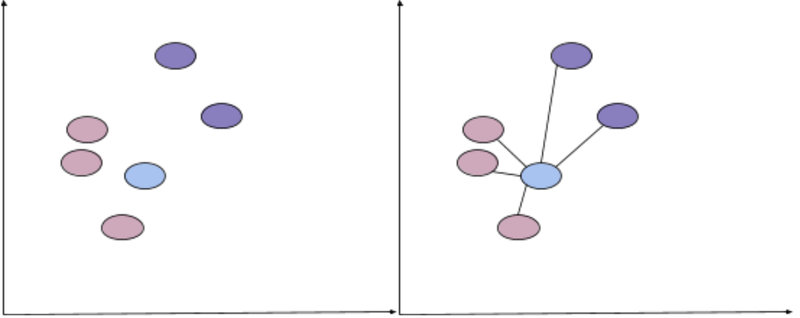What you choose k to be is important: too small a value could mean noise and inaccurate results, whereas too large a value is not feasible. Because no computation is done until it's time to make a prediction, KNN is considered a lazy learning algorithm.

You may use KNN for recommendations, image recognition, and decision-making models. It takes longer on each computation than Naive Bayes because it needs to keep track of the training data and find the neighbor nodes.

5. Random Forest🌲 is a more-complicated popular ensemble ML algorithm, meaning it combines multiple ML methods or algorithms into one predictive model where the opinion of many is more accurate than the opinion of one, that can be used for both regression and classification problems. It uses an ensemble of decision trees, building a "forest" of them.

For example, given a labelled dataset (a series of points each has a color), how would a new sample (a point) be classified (assigned a color)?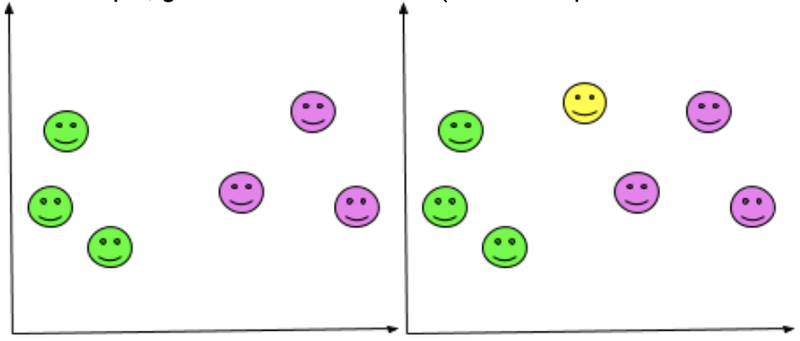What color do you think the new (yellow) point would be? Probably pink, right? We came to that decision through a mental process: given 8 points,

if `x <= 2`, the point is orange.
Otherwise, if `x >= 2.5`, it's pink

We could also be more specific and say

if `y >= 2.5 && x <= 2`, the point is orange.
Otherwise, if `y >= 2.5 && x <= 2.5`, the point is pink.

Each conditional is a branch leading to a decision: is the point orange or pink?

That was a decision tree. To classify a new data point, random forest uses multiple decision trees to take something of a vote from each tree, combines the outcome, and comes to a conclusion according to the majority consensus. To make predictions, the new data point starts at the top of the tree with the root node and traverses down the branches of "if...then" conditionals. The branch traversals occur, repeating that same decision process, until branching must stop at a leaf node. The leaf endpoint represents the final output of either a predicted class or value.Random forest uses multiple random decision trees for higher accuracy, combining its results into one final result. Predictions are made according to the average for regression problems and majority vote among trees for classification problems.

### What's Next

With these popular new machine learning algorithms in your toolkit, you should be better prepared to perform classification and regression. Let me know in the comments or online what you're working on--I can't wait to see what you build.

Rate this post
Authors
•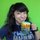Lizzie Siegle
Not ready yet? Talk to an expert.

## Thanks for rating this post!

We are always striving to improve our blog quality, and your feedback is valuable to us. How could this post serve you better?